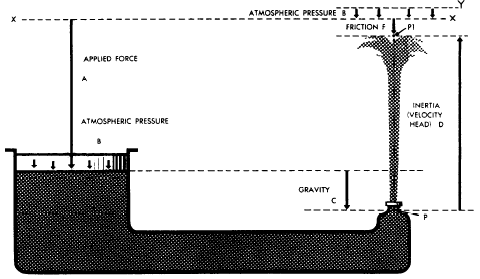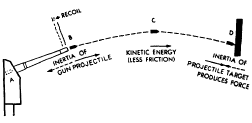Custom SearchFACTORS INVOLVED IN FLOW

An understanding of the behavior of fluids in motion, or solids for that matter, requires an understanding of the term inertia. Inertia is the term used by scientists to describe the property possessed by all forms of matter that makes the matter resist being moved if it is at rest, and likewise, resist any change in its rate of motion if it is moving.

The basic statement covering inertia is Newtons first law of motioninertia. Sir Isaac Newton was a British philosopher and mathematician. His first law states: A body at rest tends to remain at rest, and a body in motion tends to remain in motion at the same speed and direction, unless acted on by some unbalanced force. This simply says what you have learned by experiencethat you must push an object to start it moving and push it in the opposite direction to stop it again.

A familiar illustration is the effort a pitcher must exert to make a fast pitch and the opposition the catcher must put forth to stop the ball. Similarly, considerable work must be performed by the engine to make an automobile begin to roll; although, after it has attained a certain velocity, it will roll along the road at uniform speed if just enough effort is expended to overcome friction, while brakes are necessary to stop its motion. Inertia also explains the kick or recoil of guns and the tremendous striking force of projectiles.

Inertia and Force

To overcome the tendency of an object to resist any change in its state of rest or motion, some force that is not otherwise canceled or unbalanced must act on the object. Some unbalanced force must be applied whenever fluids are set in motion or increased in velocity; while conversely, forces are made to do work elsewhere whenever fluids in motion are retarded or stopped.

There is a direct relationship between the magnitude of the force exerted and the inertia against which it acts. This force is dependent on two factors: (1) the mass of the object (which is proportional to its weight), and (2) the rate at which the velocity of the object is changed. The rule is that the force in pounds required to overcome inertia is equal to the weight of the object multiplied by the change in velocity, measured in feet per second, and divided by 32 times the time in seconds required to accomplish the change. Thus, the rate of change in velocity of an object is proportional to the force applied. The number 32 appears because it is the conversion factor between weight and mass.

There are five physical factors that can act on a fluid to affect its behavior. All of the physical actions of fluids in all systems are determined by the relationships of these five factors to each other. Summarizing, these five factors are as follows:

1. Gravity, which acts at all times on all bodies, regardless of other forces

2. Atmospheric pressure, which acts on any part of a system exposed to the open air

3. Specific applied forces, which mayor may not be present, but which, in any event, are entirely independent of the presence or absence of motion

4. Inertia, which comes into play whenever there is a change from rest to motion or the opposite, or whenever there is a change in direction or in rate of motion

5. Friction, which is always present whenever there is motion

Figure 2-16 illustrates a possible relationship of these factors with respect to a particle of fluid (P) in a system. The different forces are shown in terms of head, or in other words, in terms of vertical columns of fluid required to provide the forces. At the particular moment under consideration, a particle of water (P) is being acted on by applied force (A), by atmospheric pressure (B), and by gravity (C) produced by the weight of the fluid standing over it. The particle possesses sufficient inertia or velocity head to rise to level P1, since head equivalent to F was lost in friction as P passed through the system. Since atmospheric pressure (B) acts downward on both sides of the system, what is gained on one side is lost on the other.

If all the pressure acting on P to force it through the nozzle could be recovered in the form of elevation head, it would rise to level Y. If account is taken of the balance in atmospheric pressure, in a frictionless system, P would rise to level X, or precisely as high as the sum of the gravity head and the head equivalent to the applied force.

Kinetic Energy

It was previously pointed out that a force must be applied to an object in order to give it a velocity or to increase the velocity it already has. Whether the force begins or changes velocity, it acts over a certain distance. A force acting over a certain distance is work. Work and all forms into which it can be changed are classified as energy. Obviously then, energy is required to give an object velocity. The greater the energy used, the greater the velocity will be.

Disregarding friction, for an object to be brought to rest or for its motion to be slowed down, a force opposed to its motion must be applied to it. This force also acts over some distance. In this way energy is given up by the object and delivered in some form to whatever opposes its continuous motion. The moving object is therefore a means of receiving energy at one place (where its motion is increased) and delivering it to another point (where it is stopped or retarded). While it is in motion, it is said to contain this energy as energy of motion or kinetic energy.

Since energy can never be destroyed, it follows that if friction is disregarded the energy delivered to stop the object will exactly equal the energy that was required to increase its speed. At all times the amount of kinetic energy possessed by an object depends on its weight and the velocity at which it is moving.Figure 2-16.Physical factors governing fluid flow.

The mathematical relationship for kinetic energy is stated in the rule: "Kinetic energy in foot-pounds is equal to the force in pounds which created it, multiplied by the distance through which it was applied, or to the weight of the moving object in pounds, multiplied by the square of its velocity in feet per second, and divided by 64.s"

The relationship between inertia forces, velocity, and kinetic energy can be illustrated by analyzing what happens when a gun fires a projectile against the armor of an enemy ship. (See fig. 2-17.) The explosive force of the powder in the breach pushes the projectile out of the gun, giving it a high velocity. Because of its inertia, the projectile offers opposition to this sudden velocity and a reaction is set up that pushes the gun backward (kick or recoil). The force of the explosion acts on the projectile throughout its movement in the gun. This is force acting through a distance producing work. This work appears as kinetic energy in the speeding projectile. The resistance of the air produces friction, which uses some of the energy and slows down the projectile. Eventually, however, the projectile hits its target and, because of the inertia, tries to continue moving. The target, being relatively stationary, tends to remain stationary because of its inertia. The result is that a tremendous force is set up that either leads to the penetration of the armor or the shattering of the projectile. The projectile is simply a means of transferring energy, in this instance for destructive purpose, from the gun to the enemy ship. This energy is transmitted in the form of energy of motion or kinetic energy.

A similar action takes place in a fluid power system in which the fluid takes the place of the projectile. For example, the pump in a hydraulicFigure 2-17.Relationship of inertia, velocity, and kinetic energy.

system imparts energy to the fluid, which overcomes the inertia of the fluid at rest and causes it to flow through the lines. The fluid flows against some type of actuator that is at rest. The fluid tends to continue flowing, overcomes the inertia of the actuator, and moves the actuator to do work. Friction uses up a portion of the energy as the fluid flows through the lines and components.Integrated Publishing, Inc. - A (SDVOSB) Service Disabled Veteran Owned Small Business